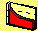3.4 CHAPTER 3: EXERCISES

1. How is portfolio risk quantified? Discuss some advantages and disadvantages associated with using this measure to quantify risk.

a) What is a variance-covariance matrix of returns?

In a variance-covariance matrix of returns:

b) Define statistically the diagonal terms (i.e., the contents when the row and column number of the variance-covariance matrix are equal).

c) Define statistically the off-diagonal terms (i.e., the contents when the row and column number of the variance-covariance matrix are not equal).

3. Suppose the variance-covariance matrix of returns for three firms, XYZ, ABC, and DEF companies, is provided as follows:

 XYZ ABC DEF XYZ 0.005 0.012 0.003 ABC 0.012 0.034 -0.005 DEF 0.003 -0.005 0.012

a). Compute the portfolio variance for an equally weighted portfolio that contains XYZ and ABC.

b) Compute the portfolio variance for an equally weighted portfolio that contains all three firms (XYZ, ABC, and DEF).

4. Using CAPM Tutor and the dataset SP20.DAT, study the effects of portfolio size on portfolio variance, as follows. The dataset provides the variance-covariance matrix of returns constructed from closing daily prices for the 20 largest stocks in the S&P 100.

a) Select five stocks from the twenty, and record the portfolio variance.

b) Add five stocks to the portfolio selected in part a). Record the variance of this 10-stock portfolio.

c) Add five more stocks to the portfolio created in part b). Record the variance of this 15-stock portfolio.

d) From parts a), b) and c) plot the relationship between portfolio size and portfolio variance.

e) Explain why increasing the number of stocks generally reduces portfolio variance.

(You may want to experiment with different selections of stocks).

5. Construct a numerical example in which adding one stock to a portfolio increases the variance of the naively diversified portfolio.

6. Explain why the "average" covariance is what matters in a portfolio with a large number of stocks.

7. In CAPM Tutor you have available three "industry" datasets, ENERGY.DAT, FINANCE.DAT, PETRO.DAT, and a file called INDUS.DAT. The first three datasets each contain a variance-covariance matrix of daily returns for 5 firms within the industry, while INDUS.DAT has information on industry-specific mutual funds constructed from the 5 firms.

Each firm in the dataset belongs to the S&P100 stock index.

a) For each industry, record the smallest portfolio variance you can achieve by diversifying naively among the stocks within the industry.

b) Using INDUS.DAT, record the smallest portfolio variance you can achieve by diversifying naively across industries.

c) Explain why you may not want to diversify naively across the three industries..

8) The dataset SP20.DAT contains the variance-covariance matrix of daily returns for the top 20 stocks in the S&P 100 index. Read this data set into the naive diversification chapter of CAPM Tutor and use the include/exclude option to choose subsets of 4 firms from the top 20 firms.

a). Choose four firms so that the minimum portfolio variance reduces as the naive portfolio size increases from 1 to 2 to 3 to 4 firms. Record and plot the variance for each firm and the minimum portfolio variance for each size.

b) Choose 4 firms so that the minimum portfolio variance is reduced as the naive portfolio size increases from 1 to 2, then increases as the portfolio size increases to 3 and then is reduced again by adding the fourth security. Record and plot the variance for each firm and the minimum portfolio variance for each size.

c) Import the file SP20.DAT into any spreadsheet. This is a 20X20 matrix where the rows 1-20 and cols 1-20 correspond to the top 20 firms in the S&P100 described in DATASETS.TXT. A complete description of SP20.DAT is contained in the text file DATASETS.TXT. From this big matrix construct the variance-covariance matrix of returns for the four firms you selected in part a) and similarly, construct the variance covariance matrix of returns for the four firms that you have selected in part b).

d) Using the two variance-covariance matrices that you constructed in part c) explain why you obtain the different shapes when plotting the minimum portfolio variance by size in parts a) and b).

previous topic

next topic